# Geometry¶

Basic construction elements are simple geometry types, that are used for shape definition of structural members and geometrical object. With defining the further attributes to these elements from the lists Structural analysis elements, Supports and hinges and Loads the complete structural model is created. All values refer to the list StrucutralPointConnection.

## Geometry types¶

Geometry type

Type definition

Insertion data explanation

SAF geometry strings

Notes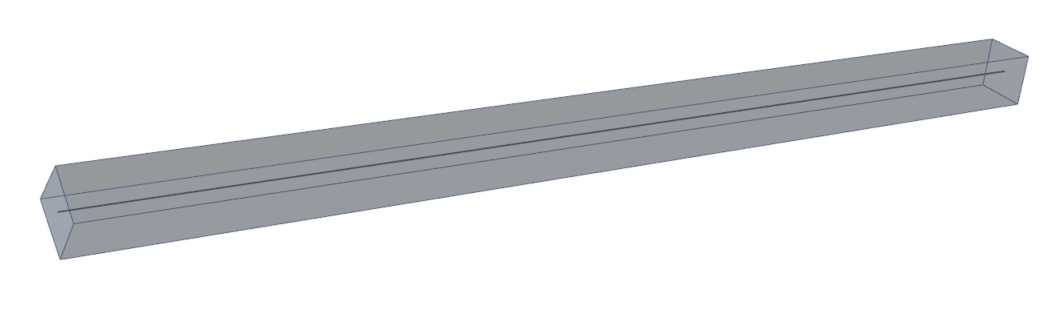Line

Straight-line between two nodes

Start point , End point

N1;N2

Line

-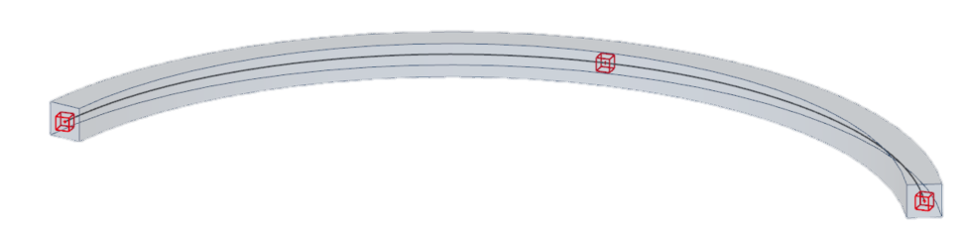Circular Arc

Arch defined with 3 nodes

Start point, Intermediate point, End point

N3;N4;N5

Circular Arc

-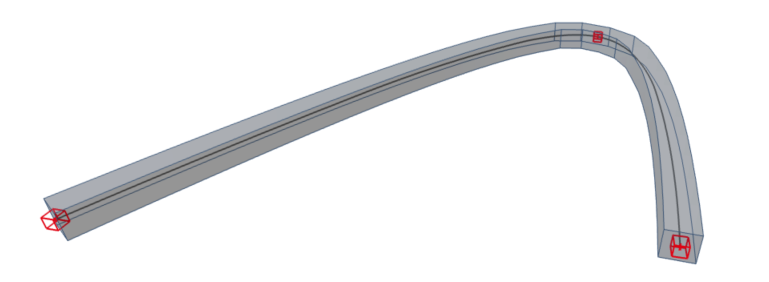Parabolic Arc

Parabolic arch defined with 3 nodes

Start point, Intermediate point, End point

N6;N7;N8

Parabolic Arc

-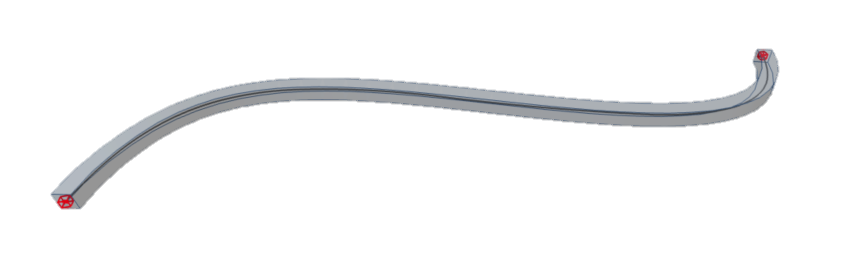Bezier

Cubic Bezier curve

Start point, 2nd point of control polygon (vertex), 3rd point of control polygon (vertex), End point

N9;Vertex_B1_1;

Vertex_B1_2;N10

Bezier

N9 and N10 stands for start and end node

Vertex_B1_1, Vertex_B1_2 define vertexes of bezier curve

All values refers to list StrucutralPointConnection

Bezier curve is parabolic, when 2nd and 3rd control points are the identical (values of coordinates are the same)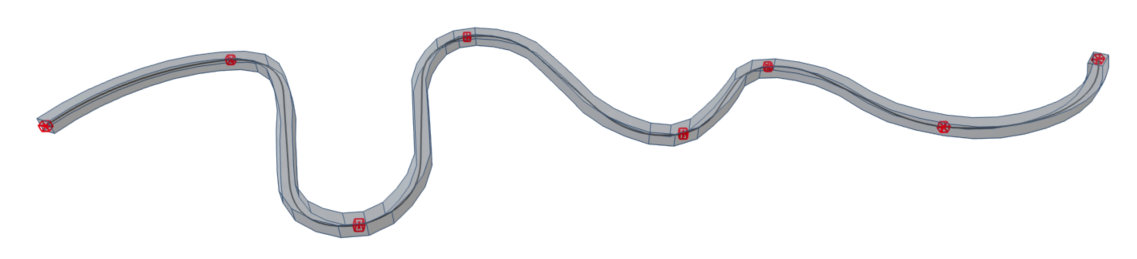Spline

Curved line defined by polynomial function

Start point, Set of mid points, End point

N11;N12;N13;N14; N15;N16;N17;N18

Spline-8

“Spline-#” where “#” stands for number of nodes defining the spline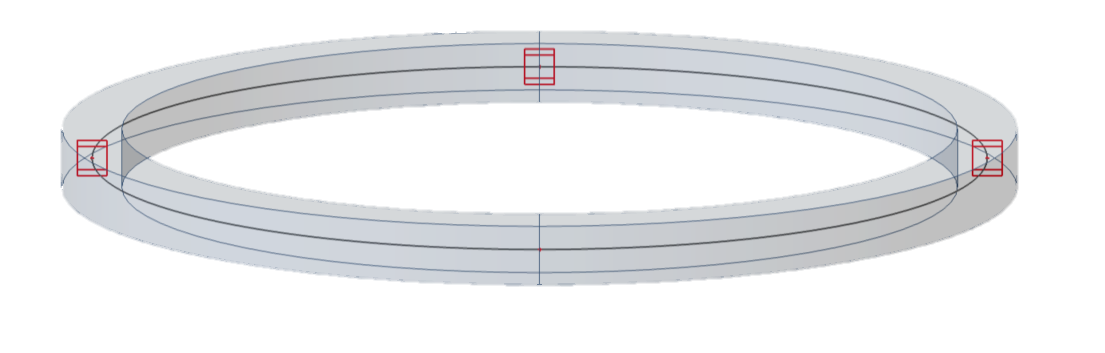Circle

Circle

Center Point, Point on the perimeter

N36;N37

Or

Three point on perimeter

N36;N37;N38

Circle and Point

or

Circle by 3 points

Circle is not valid to define StrucutralCurveMember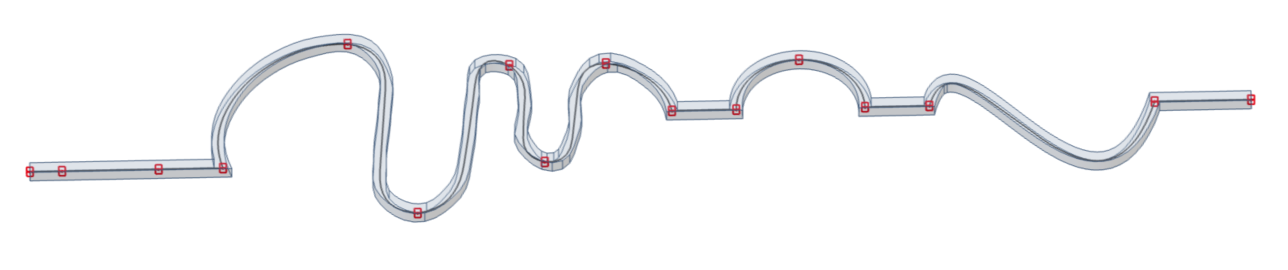Polyline

Combination of in nodes connected geometric types

List of nodes

N21;N22;N23;N24;N25; N26;N27;N28;N29;N30; N31;N32;N33;Vertex_B1_1; VertexB_1_2;N34;N35

Line;Line;Spline-7;Line;Circular Arc;Line;Bezier;Line

Detail explanation can be found in notes below